# Stat-Xplore : Derivations

Derivations allow you to create new calculated items within a table. For example, you can add together values in other columns, or use mathematical and statistical functions.

For example, in the following table a derivation has been added to calculate the total number of customers who are located in the North (the total number of Universal Credit claimants in Scotland, the North East and the North West):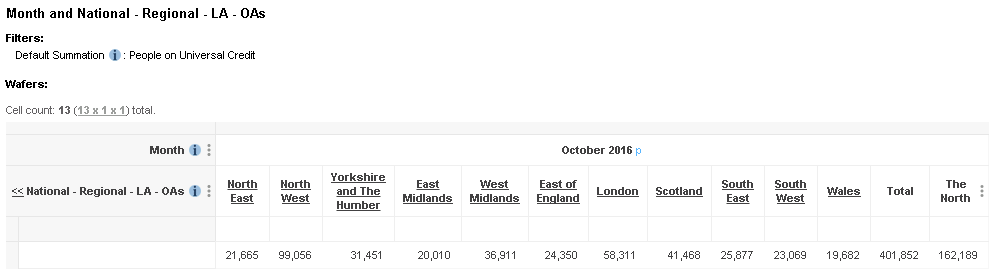Derivations are saved if you save the table in Stat-Xplore, and will remain in the table as long as you do not remove the field they are derived from, or change the set of field values from the derived field that are in the table. For example, the above derivation for Region would automatically be removed if you removed the field value North East from the table or added a field value that didn't already exist in the table.

Changes to other fields do not affect derivations (for example adding or remove field values from Gender would not affect the derivation on Region). You can also move the derived field to a different axis without losing your derivations.

### Create a Derivation

To create a derivation, click the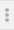menu option in the table next to the name of the field, and select the Add Derivation option: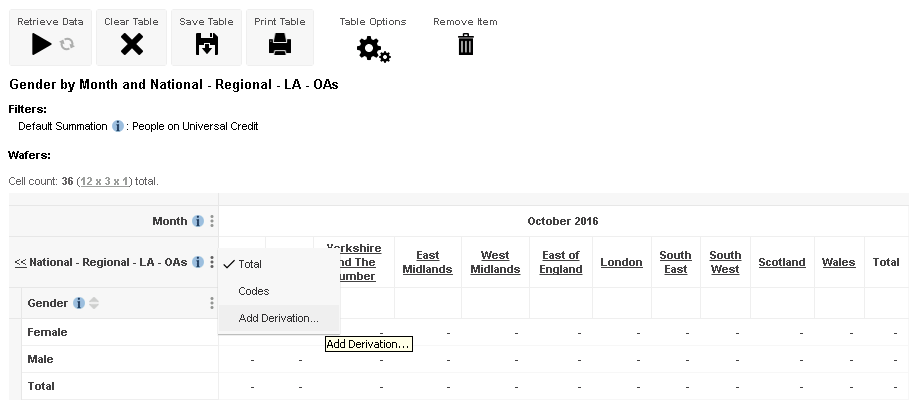The Define Derivation dialog displays: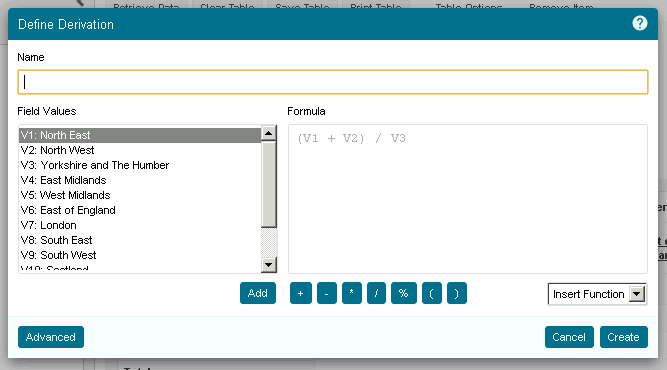To create the derivation:

1. In the Name field, enter a name for the derivation. This name will be displayed in the table.
2. Use the Formula field to enter your formula.
• You can either type the formula in manually, or use the buttons, drop-down list of functions and the Field Values list to select and add field items and mathematical and statistical functions.
• You can double-click a field value in the list to add it to the formula. Alternatively, select one or more field items (hold down Shift or Ctrl to make multiple selections) and use the Add button (this will add the sum of the selected field items to the formula).
• To refer to a field value in your formula, you must use the value codes (in this example, the code V1 represents North East, V2 represents North West, and so on).
• To add one of the functions, you can either type it manually into the formula, or select from the drop-down list as follows (see below for a description of the available functions):
1. In the Field Values list, select the item or items you want to use with the function.
2. Select the function from the drop-down list. Some of the functions take one field value as an argument, while others take two or more; some of the functions will be greyed out if the number of selected items does not match the number of arguments they take.

For example: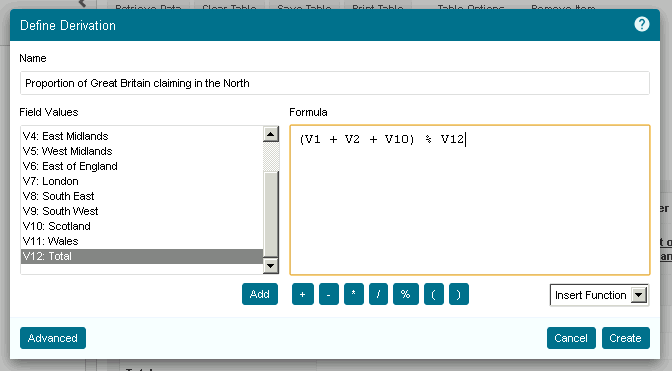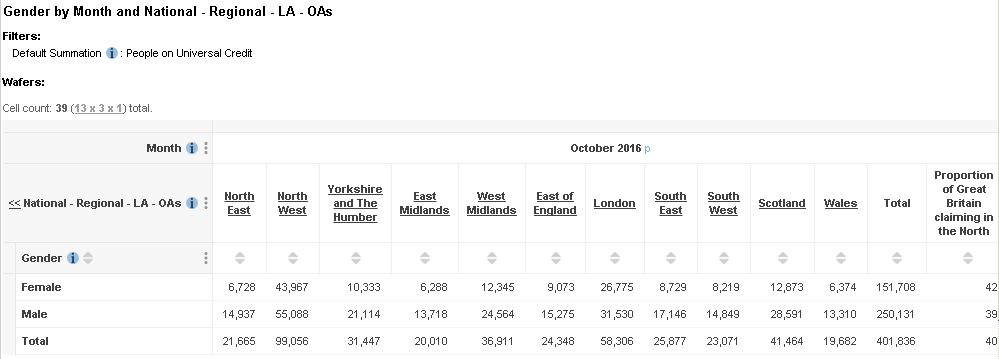### Derivation Functions

In addition to the mathematical operators (such as +, -, * and /) you can also use some functions in your derivations:

`Exp`
Exponential function. For example: `Exp(V1)`
`Sqrt`
Square root. For example: `Sqrt(V1)`
`Round`
Rounds to 0 decimal places. For example: `Round(V1)`
`Ln`
Logarithm function. For example: `Ln(V1)`
`Sum`

The sum of a range of field values.

For example: `SUM(V1:V4)` calculates the sum of field values V1, V2, V3 and V4.

`Forecast`

A forecast of a future or past value. The forecast is based on a trendcast algorithm that uses linear regression to extrapolate forwards and backwards.

The function takes 2 arguments, separated by a semi-colon:

• The range of values to use in the forecast. For example: `V1:V5`
• The period to forecast for. You can use a negative value to forecast for an earlier period, and you can use a decimal value to forecast for a partial period.

For example:

 `Forecast(V1:V5;1)` Forecast the next item in the sequence after V1 to V5. If V1 to V5 represent the years 2011-2015, then this forecasts the result for 2016. ` Forecast(V1:V5;2)` Forecast the next but one item in the sequence after V1 to V5. If V1 to V5 represent the years 2011-2015, then this forecasts the result for 2017. `Forecast(V1:V5;-1)` Forecast the previous period before the start of the range V1 to V5. If V1 to V5 represent the years 2011-2015, then this forecasts the result for 2010.

### Edit a Derivation

If you want to make changes to a derivation after you have created it, click the menu next to the item in the table and select Edit Derivation.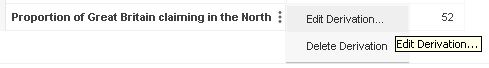Stat-Xplore displays the Define Derivation dialog, where you can make changes. When you have finished, click Save to apply the changes and save your updates to the table.

### Create/Edit a Derivation: Advanced Options

When you are creating or editing a derivation, you can click the Advanced option at the bottom of the Define Derivation dialog to access some advanced options: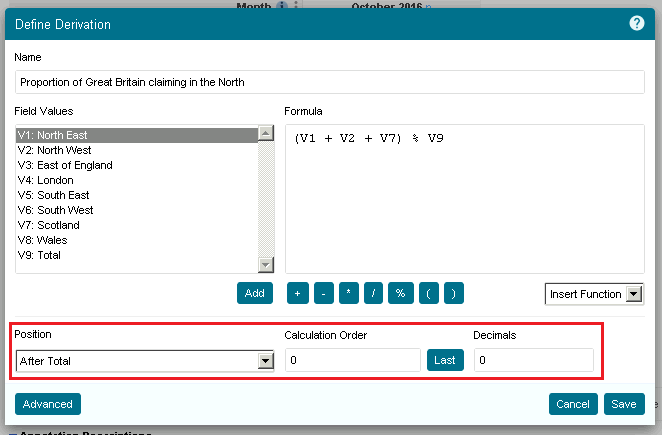The advanced options are as follows:

Position The position of the derivation within the table. By default, derivations are added as the last item in the row or column. Select an option from the drop-down list to move the derivation to either the start, end or after a specific field value. Set the order of evaluation for the derivation. This can be important if you have multiple derivations in a table (for example, if you have derivations in both rows and columns, what should Stat-Xplore display in the cell where the two derivations intersect?). It can also be important if you are calculating percentages of fields and using totals. Fields with a lower number are evaluated first. Either enter a number or click Last to set the derivation to be evaluated last out of all currently defined derivations (when you click  Last, Stat-Xplore populates the Calculation Order field with an evaluation number that is higher than any other currently defined derivation). Enter the number of decimal places to display for this derivation.

### Delete a Derivation

To remove a derivation, click the menu next to the item in the table and select Delete Derivation.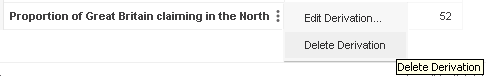## Attachments: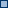SW2-DerivationsExample.png (image/png)SW2-AddDerivation.png (image/png)SW2-Table-Menu.png (image/png)SW2-DefineDerivation.png (image/png)SW2-DefineDerivation-Example.png (image/png)SW2-DefineDerivation-Example.png (image/png)SW2-DerivationInTable.png (image/png)SW2-EditDerivation.png (image/png)SW2-DerivationAdvancedOptions.png (image/png)SW2-Delete-Derivation.png (image/png)SW2-DefineDerivation.png (image/png)SW2-DefineDerivation-Example.png (image/png)SW2-DerivationAdvancedOptions.png (image/png)SW2-DerivationDecimalMark.png (image/png)SW2-DerivationDecimalMarkCorrect.png (image/png)SW2-DerivationsExample.png (image/png)SW2-AddDerivation.png (image/png)SW2-DerivationInTable.png (image/png)SW2-DerivationsExample.png (image/png)SW2-AddDerivation.png (image/png)SW2-DerivationInTable.png (image/png)SW2-EditDerivation.png (image/png)SW2-Delete-Derivation.png (image/png)